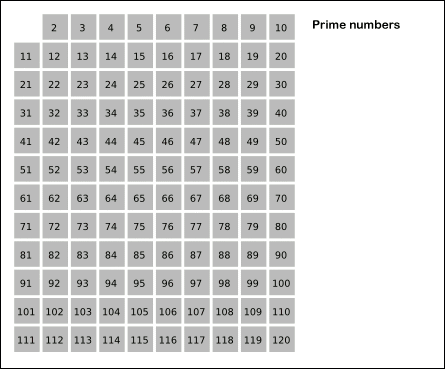ARTS 第二周
Toc
ARTS Week-2## Algorithm

204. 计数质数

输入: 10



class Solution:
def countPrimes(self, n: int) -> int:
count = 0

for i in range(n):
if self.isPrime(i):
count += 1

return count

def isPrime(self, n) -> bool:
if n < 2:
return False

if n == 2:
return True

i = 2

while i <= int(n ** 0.5):

if n % i == 0:
return False

i += 1

return Trueclass Solution:
def countPrimes(self, n: int) -> int:
if n <= 2:
return 0

arr =  * n

for i in range(2, n):

j = 2
mul = i * j

while mul < n:

arr[mul] = 0
j += 1
mul = i * j

return sum(arr[2:])


class Solution:
def countPrimes(self, n: int) -> int:
if n <= 2:
return 0

arr =  * n

for i in range(2, int(n)):
if arr[i] == 1:
arr[2*i:n:i] =  * len(arr[2*i:n:i])

return sum(arr[2:])


Github 仓库地址

## Review

《Distributed systems theory for the distributed systems engineer》

## Tip

    import functools
​
def force_async(fn):
"""
turns a sync function to async function using threads
"""
import asyncio

@functools.wraps(fn)
def wrapper(*args, **kwargs):
future = pool.submit(fn, *args, **kwargs)
return asyncio.wrap_future(future)  # make it awaitable

return wrapper
​
def force_sync(fn):
"""
turn an async function to sync function
"""
import asyncio

@functools.wraps(fn)
def wrapper(*args, **kwargs):
res = fn(*args, **kwargs)
if asyncio.iscoroutine(res):
return asyncio.get_event_loop().run_until_complete(res)
return res

return wrapper